# SSAT Upper Level Math : How to find if right triangles are similar

## Example Questions

### Example Question #1 : How To Find If Right Triangles Are Similar

If a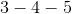right triangle is similar to a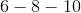right triangle, which of the other triangles must also be a similar triangle?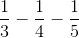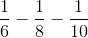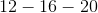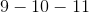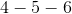Explanation:

For the triangles to be similar, the dimensions of all sides must have the same ratio by dividing the 3-4-5 triangle.

The 6-8-10 triangle will have a scale factor of 2 since all dimensions are doubled the original 3-4-5 triangle.

The only correct answer that will yield similar ratios is thetriangle with a scale factor of 4 from the 3-4-5 triangle.

The other answers will yield different ratios.

### Example Question #2 : How To Find If Right Triangles Are Similar

What is the main difference between a right triangle and an isosceles triangle?

An isosceles triangle has to have aangle and a right triangle has to haveequal, base angles.

A right triangle has to have a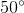angle and an isosceles triangle has to haveequal, base angles.

A right triangle has to have aangle and an isosceles triangle has to haveequal, base angles.

A right triangle has to have aangle and an isosceles triangle has to haveequal, base angles.

A right triangle has to have aangle and an isosceles triangle has to haveequal, base angles.

A right triangle has to have aangle and an isosceles triangle has to haveequal, base angles.
By definition, a right triangle has to have one right angle, or aangle, and an isosceles triangle hasequal base angles and two equal side lengths.